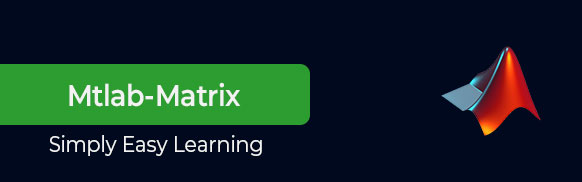# Matlab-Matrix Tutorial

MATLAB is a programming language developed by MathWorks. It started out as a matrix programming language where linear algebra programming was simple. It can be run both under interactive sessions and as a batch job. This tutorial gives you aggressively a gentle introduction of MATLAB programming language. It is designed to give students fluency in MATLAB programming language. Problem-based MATLAB examples have been given in simple and easy way to make your learning fast and effective.

# Audience

This tutorial has been prepared for the beginners to help them understand basic to advanced functionality of MATLAB. After completing this tutorial you will find yourself at a moderate level of expertise in using MATLAB from where you can take yourself to next levels.

# Prerequisites

We assume you have a little knowledge of any computer programming and understand concepts like variables, constants, expression, statements, etc. If you have done programming in any other high-level programming language like C, C++ or Java, then it will be very much beneficial and learning MATLAB will be like a fun for you.